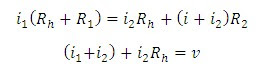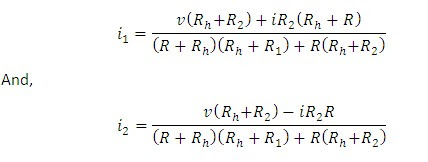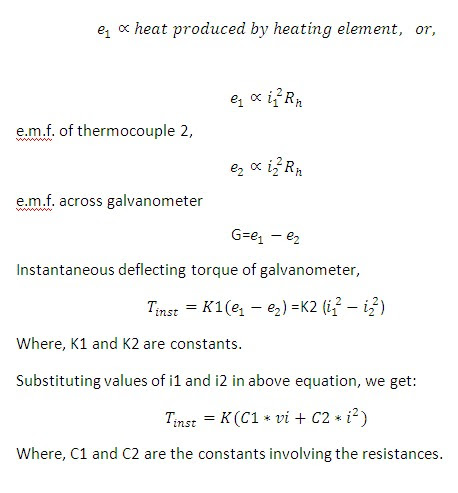# Thermal wattmeter

## Thermal wattmeter:

Following figure shows the arrangement of thermal wattmeter for measurement of power:

This wattmeter uses two similar thermocouples (1 and 2) whose outputs are connected in opposition with a galvanometer in between. Rh is the resistance of each thermocouple heating element. R is high series resistance, and between C and D is a low resistance R2 capable of carrying the load current i. the resistance R2 develops a potential difference which depends upon the load current, together with the current of one heater, and the series resistance R carries the current of both heaters.

If v be the instantaneous voltage at the load, then assuming identical thermocouples, we have:From above two equations, we get:e.m.f. of thermocouple 1,If  Rh+R  is not very different from Rh, the C2*i^2 may be neglected and T(inst.) is directly proportional to v*i or instantaneous power.

Thus, galvanometer may be calibrated to read the power.

• The commercial thermal wattmeters employ a number of thermocouples connected in the form of a chain in order to increase the output. C.T.s and P.T.s are also used with these instruments.

• For high frequency measurements careful shielding is required.

The thermal wattmeters can be used for measurement of power is several circuits and the sum of their outputs can be applied to a recording potentiometer which records the total power.

You may also like:

Electrostatic type Wattmeter

Dynamometer type wattmeter

tags:  thermal wattmeter differential thermal wattmeterthermal wattmeter with direct applications of thermal wattmeter advantages of thermal wattmeter disadvantages of thermal wattmeter

1.2.